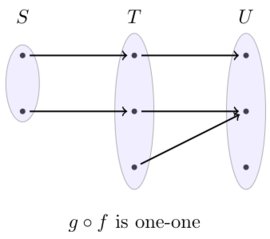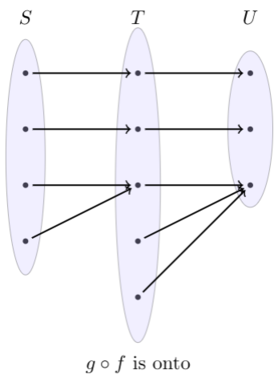# Functions

### One-to-one function

A1, 2013

For sets $$S$$ and $$T,$$ let $$f: S \rightarrow T$$ and $$g: T \rightarrow S$$ be functions such that $$f(g(x))=x$$ for each $$x$$. State which of the statements are true.

• (a) The function $$f$$ must be one-to-one.
• (b) The function $$g$$ must be one-to-one.
• (c) The function $$f$$ must be onto.
• (d) The function $$g$$ must be onto.

Solution

False-True-True-False.

If $$g\left(x_{1}\right)=g\left(x_{2}\right),$$ then $$x_{1}=f\left(g\left(x_{1}\right)\right)=f\left(g\left(x_{2}\right)\right)=x_{2},$$ so $$g$$ is one-to-one. Also $$f$$ is onto because each $$x \in T$$ is in the image of $$f,$$ namely $$x=f(g(x)) .$$ The other two statements are false, for example by constructing an $$S$$ that is a larger finite set than $$T$$.

### Composition

B1a, 2021

$$f$$ is a function defined on the domain $$S$$ and codomain $$T$$ and $$g$$ is a function defined on the domain $$T$$ and codomain $$U$$. Fill in the blanks with with one of the four options.
If $$g\circ f$$ is one-one then, $$f$$ is $$\underline{.......}$$ and $$g$$ is $$\underline{......}$$.
If $$g\circ f$$ is onto then, $$f$$ is $$\underline{.......}$$ and $$g$$ is $$\underline{......}$$.

Options:
A: One-one and onto.
B: One-one but need not to be onto.
C: Onto but need not to be one-one.
D: Need not to be one-one and need not be onto.

Solution

Ans. B-D-D-C.
Explanation. If $$g\circ f$$ is one-one, the $$f$$ must be one-one. If not, there are two elements $$x$$ and $$y$$ with $$x\neq y$$ such that $$f(x) = f(y)$$, which implies that $$g(x) = g(y)$$. A contradiction. However, $$f$$ need not be onto and $$g$$ need not be onto or one-one as the example below shows.If $$g\circ f$$ is onto, then $$g$$ must be onto since every element in $$U$$ must be reached by some element from $$S$$ via $$T$$. As the example below shows, this the only constraint.See part (b) of the question

### Asymptotes

A5, 2020

Write whether the $$3$$ functions $$\frac{x^3}{(x^2-x)}, \ \frac{(x^2-x)}{x^3},\ \frac{(x^3-x)}{(x^3+x)}$$ have horizontal asymptotes, vertical asymptotes and removable discontinuities.

Solution

#### How to find a horizontal asymptote?

Let us consider the case when the given function is of the form: $f(x) = \frac{a_1x^m+a_2x^{m-1}+\cdots+a_m}{b_1x^n+b_2x^{n-1}+\cdots+b_n}$
• If $$m> n$$, then there is no horizontal asymptote.
• If $$m< n$$, then $$y=0$$ a horizontal asymptote.
• If $$m=n$$, then $$y=a_1/b_1$$ a horizontal asymptote.
We can apply this directly to the given functions. The first function has no horizontal asymptotes. The second and third functions have $$y=0$$ and $$y=1$$ as their horizontal asymptotes, respectively.

#### How to find a vertical asymptote?

Vertical asymptotes occur at those points where the denominator is zero and the numerator is non-zero.

The first two functions have $$x=1$$ and $$x=0$$ as their vertical asymptotes. The denominator is always positive for the third function, so there are no vertical asymptotes for this function.

#### Removable discontinuities

In all the functions, the term $$x$$ can be factored out from the numerator and the denominator. Hence, $$x=0$$ is a removable discontinuity for all the functions.

### Constrained function

B4, 2020

i) A continuous function $$f(x)$$ has the property that $$f(x^2)=f(x)^2.$$ If the domain of $$f$$ is $$[0,1]$$ and $$f(0)\neq 0,$$ then show that $$f$$ is unique and find $$f.$$

Solution

Since $$f(0)$$ is non-zero, $$f(0)=f(0)^2 \implies f(0)=1$$. Since $$f(x) = f(\sqrt{x})^2$$, the range of $$f$$ is non-negative. We will show that $$f(x)=1$$.

Let $$p\in (0,1)$$ be an arbitrary point and $$f(p) = q$$. \begin{align} f(p^2) &= f(p)^2 = q^2 \\ f(p^4) &= q^4 \\ &\vdots \\ f(p^{2^n}) &= q^{2^n} \end{align} Since $$|p|< 1$$ the sequence $${p^{2^n}}$$ converges to 0 as $$n\rightarrow \infty$$. Since the function is continuous: $f(0) = 1 = \lim_{n\rightarrow \infty} q^{2^n}$ The sequence $$q^{2^n}$$ must converge to 1. This is possible only if $$q=1$$. By continuity, $$f(1)=1$$ too. Therefore, the conditions imply that $$f$$ is unique and that $$f(x)=1$$.

ii) Consider the same property of $$f,$$ but the domain of the function being $$(0,\infty).$$ Show that either $$f(x)=0\ \forall x$$ or $$f(x)\neq 0$$ $$\forall$$ $$x.$$

Solution

The proof is similar to the previous proof. For $$x=1$$ we have: $f(1^2) = f(1)^2 \implies f(1)(f(1)-1) = 0$ So $$f(1)$$ is either 0 or 1.

Lemma. If $$f(1)=0$$, then $$f(x)=0$$.
Proof. For a contradiction, let us say there exists a point $$p$$ such that $$f(p)=q$$, where $$q>0$$. \begin{align} f(\sqrt{p})^2 &= f(p) = q \\ f(\sqrt{p}) &= \sqrt{q} \\ f(p^{ 1/2^n } ) &= q^{1/2^n} \\ \lim_{n\rightarrow \infty} f(p^{ 1/2^n } ) &= q^{1/2^n} \\ f(1) &= 1 \;\;\;\; \mbox{ (a contradiction) }\;\; \square \end{align}

Lemma. If $$f(1)=1$$, then $$f(x) \neq 0 \;\forall x$$.
Proof. For a contradiction, let us say there exists a point $$p$$ such that $$f(p)=0$$. \begin{align} f(\sqrt{p})^2 &= f(p) = 0 \\ f(\sqrt{p}) &= 0 \\ f(p^{ 1/2^n }) &= 0 \;\;\;\;\mbox{ (by induction)}\\ \lim_{n\rightarrow \infty} f(p^{ 1/2^n } ) &= 0 \\ f(1) &= 0 \;\;\;\; \mbox{ (a contradiction) }\;\; \square \end{align}

iii) Show that there exist infinitely many continuous functions $$f(x)$$ with the same property and with domain $$(0,\infty)$$ such that $$\int_0^{\infty}f(x)dx<1.$$

Solution

For any $$p>4$$, the following function satisfies the conditions: $f(x) = \left\{\begin{array}{lr} x& \text{for } 0 < x \leq 1\\ x^{-p} & \text{for } 1 < x < \infty \\ \end{array} \right.$

\begin{align} \int_0^{\infty}f(x)dx &= \int_0^{1}x dx + \int_1^{\infty} x^{-p} dx \\\\ &= \left. \frac{x^2}{2} \right \rvert_{0}^{1} \; +\; \left. \frac{x^{-p+1}}{-p+1} \right \rvert_{1}^{\infty} \\\\ &= \frac{1}{2} + \frac{1}{p-1} \\\\ &< \frac{5}{6} \;\;\;\mbox{ since }\; p>4 \end{align}

### Surjective if and only if injective

B11, 2011

A function $$f$$ from a set $$X$$ to itself satisfies $$f^{m}=f^{n}$$ for fixed positive integers $$m$$ and $$n$$ with $$m> n$$. The notation $$f^{n}$$ stands for $$f \circ f \circ \cdots \circ f(n$$ times). Show that $$f$$ is one-to-one (injective) if and only if $$f$$ is onto (surjective).

Solution

Claim 1: If $$f$$ is one-to-one, then it is onto.

Proof: Let $$m=n+k$$. $f^m(x) = f^n(x) \implies f^n( f^k(x) ) = f^n(x) \implies f^k(x) = x \text{ for all } x$ The second implication follows since $$f(p)=f(q)$$ implies that $$p=q$$ for a one-to-one function. Since $$f^k$$ is an identity function, its range must be $$X$$. So the range of $$f$$ must also be $$X$$, which proves the claim. $$\;\square$$

Claim 2: If $$f$$ is onto, then it is one-to-one.

Proof: We prove by contradiction. Assume that $$f$$ is onto but not one-to-one. So there must be an $$x$$ such that $$f(a_1) = f(b_1) = x$$ for some $$a_1\neq b_1$$. Since $$f$$ is onto we should be able to find two numbers $$a_2$$ and $$b_2$$ such that $f(a_2) = a_1 \text{ and } f(b_2) = b_1$ Also, $$a_1\neq b_1 \implies a_2\neq b_2$$. It follows that: $f^2(a_2) = x \text{ and } f^2(b_2) = x$ In general, for every natural number $$t>1$$, we should be able to find two different numbers $$a_t,b_t$$ with the property that $$f^t(a_t) = f^t(b_t) = x$$ and $$f(a_t) = a_{t-1} \text{ and } f(b_t) = b_{t-1}$$. In particular, consider the numbers $$a_m$$ and $$b_m$$, where $$f^m(a_m) = f^m(b_m) = x$$. Due to the condition $$f^m=f^n$$, we have: $x = f^m(a_m) = f^n(a_m) = a_{m-n} = a_k$ $x = f^m(b_m) = f^n(b_m) = b_{m-n} = b_k$ This implies that $$a_k=b_k$$, which is a contradiction.Hence, the function $$f$$ is one-to-one if $$f$$ is onto. $$\;\quad\square$$

### Continuity of two functions

A10, 2017

Consider the following function: $f(x)=\left\{\begin{array}{ll} x^{2} \cos \left(\frac{1}{x}\right), & x \neq 0 \\ a & x=0 \end{array}\right.$

(a) Find the value of $$a$$ for which $$f$$ is continuous.

(b) $$f^{\prime}(0)$$

(c) $$\lim_{x \rightarrow 0} f^{\prime}(x)$$

Solution

(a) $$\cos \left(\frac{1}{x}\right)$$ is sandwiched between -1 and $$1,$$ so $$\lim_{x \rightarrow 0} f(x)=0=a$$ makes $$f$$ continuous.

(b) Now $$f^{\prime}(0)=\lim_{h \rightarrow 0} \frac{h^{2} \cos \left(\frac{1}{h}\right)-0}{h}=\lim_{h \rightarrow 0} h \cos \left(\frac{1}{h}\right)$$ which is similarly 0.

(c) For nonzero $$x,$$ calculate $$f^{\prime}(x)=2 x \cos \left(\frac{1}{x}\right)+\sin \left(\frac{1}{x}\right),$$ so $$\lim_{x \rightarrow 0} f^{\prime}(x)$$ does not exist $$\operatorname{as} \lim_{x \rightarrow 0} 2 x \cos \left(\frac{1}{x}\right)=0$$ and $$\lim_{x \rightarrow 0} \sin \left(\frac{1}{x}\right)$$ does not exist.